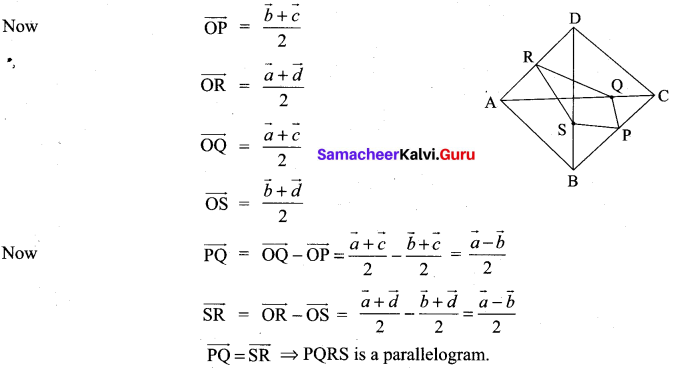# Samacheer Kalvi 11th Maths Solutions Chapter 8 Vector Algebra – I Ex 8.1

## Tamilnadu Samacheer Kalvi 11th Maths Solutions Chapter 8 Vector Algebra – I Ex 8.1

Question 1.
Represent graphically the displacement of
(i) 45 cm 30 ° north of east
(ii) 80 km, 60° south of west
Solution:
(i) 45 cm 30 0 north of east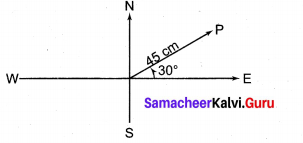(ii) 80 km 60° south of west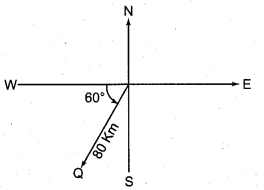Question 2.
Prove that the relation R defined on the set V of all vectors by $$\vec{a}$$ R $$\vec{b}$$ if $$\vec{a}=\vec{b}$$ is an equivalence relation on V.
Solution:
$$\vec{a}$$ R $$\vec{b}$$ is given as $$\vec{a}=\vec{b}$$.
(i) $$\vec{a}$$ = $$\vec{a}$$ ⇒ $$\vec{a}$$ R $$\vec{a}$$
(i.e.,) the relation is reflexive.

(ii) $$\vec{a}=\vec{b}$$ ⇒ $$\vec{b}$$ = $$\vec{a}$$
(i.e.,) $$\vec{a}$$ R $$\vec{b}$$ – $$\vec{b}$$ R $$\vec{a}$$
So, the relation is symmetric.

(iii) $$\vec{a}=\vec{b} ; \vec{b}=\vec{c} \Rightarrow \vec{a}=\vec{c}$$
(i.e„) $$\vec{a}$$ R $$\vec{b}$$ ; $$\vec{b}$$ R $$\vec{c}$$ ⇒ $$\vec{a}$$ R $$\vec{c}$$
So the given relation is transitive
So, it is an equivalence relation.

Question 3.
Let $$\vec{a}$$ and $$\vec{a}$$ be the position vectors of the points A and B. Prove that the position vectors of the points which trisects the line segment AB are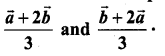Solution: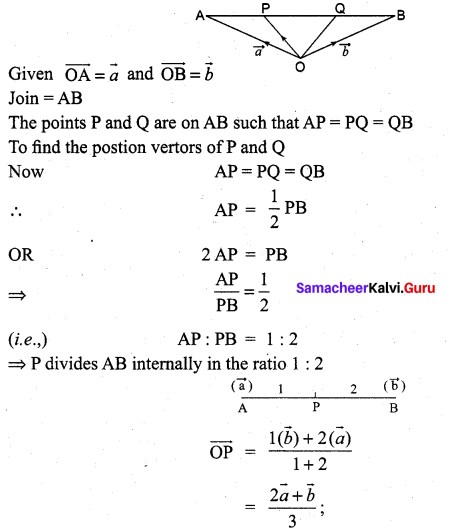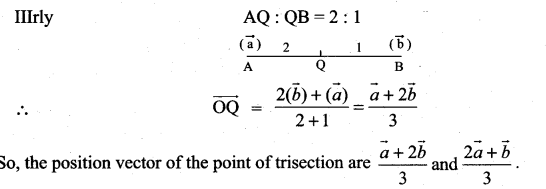Question 4.
If D and E are the midpoints of the sides AB and AC of a triangle ABC, prove that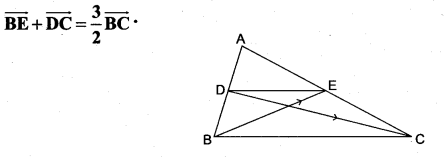Solution: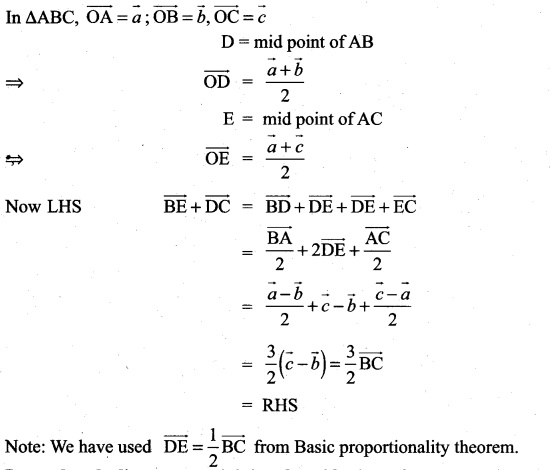Question 5.
Prove that the line segment joining the midpoints of two sides of a triangle is parallel to the third side whose length is half of the length of the third side.
Solution: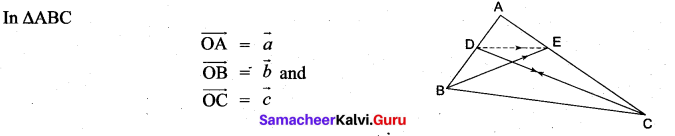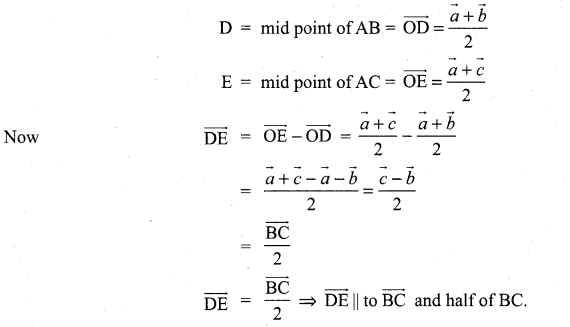Question 6.
Prove that the line segments joining the midpoints of the adjacent sides of a quadrilateral form a parallelogram.
Solution: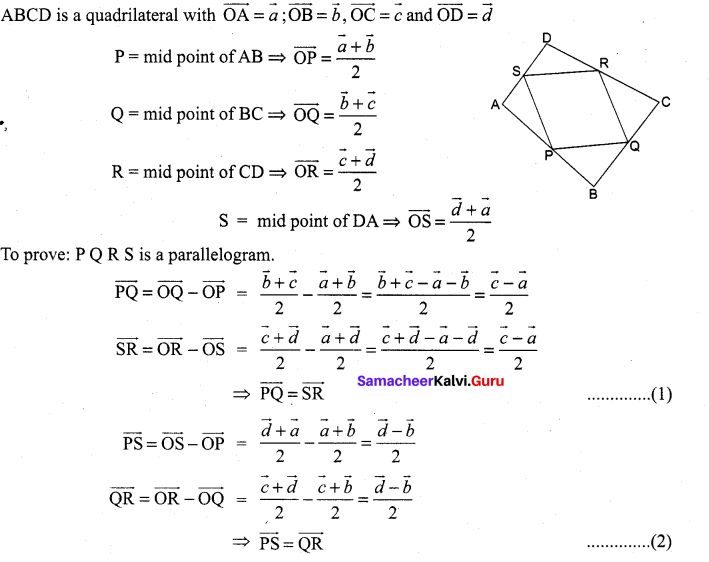In a quadrilateral when opposite sides are equal and parallel it is a parallelogram So, PQRS is a parallelogram, from (1) and (2).

Question 7.
If $$\vec{a}$$ and $$\vec{b}$$ represent a side and a diagonal of a parallelogram, find the other sides and the other diagonal.
Solution:
OABC is a parallelogram where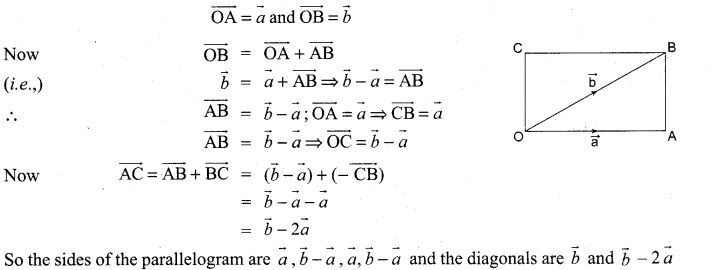Question 8.
If $$\overrightarrow{\mathrm{PO}}+\overrightarrow{\mathrm{OQ}}=\overrightarrow{\mathrm{QO}}+\overrightarrow{\mathrm{OR}}$$, prove that the points P, Q, R are collinear.
Solution: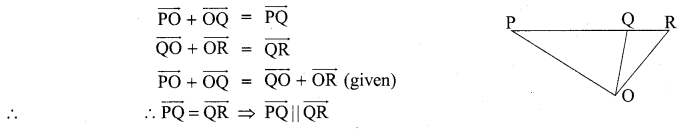But Q is a common point.
⇒ P, Q, R are collinear.

Question 9.
If D is the midpoint of the side BC of a triangle ABC, prove that $$\overrightarrow{\mathbf{A B}}+\overrightarrow{\mathbf{A C}}=2 \overrightarrow{\mathbf{A D}}$$
Solution:
D is the midpoint of ∆ ABC.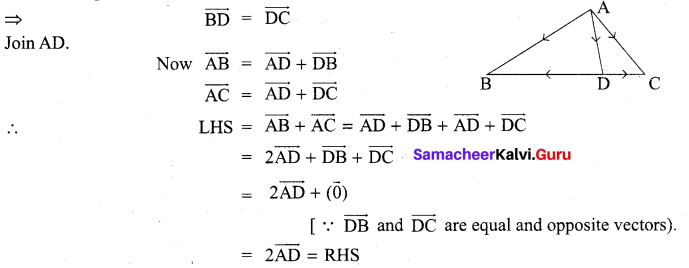Question 10.
If G is the centroid of a triangle ABC, prove that $$\overrightarrow{\mathrm{GA}}+\overrightarrow{\mathrm{GB}}+\overrightarrow{\mathrm{GC}}=0$$
Solution:
For any triangle ABC,
$$\overrightarrow{\mathrm{GA}}+\overrightarrow{\mathrm{GB}}+\overrightarrow{\mathrm{GC}}=0$$
Now G is the centroid of ∆ABC, which divides the medians (AD, BE and CF) in the ratio 2 : 1.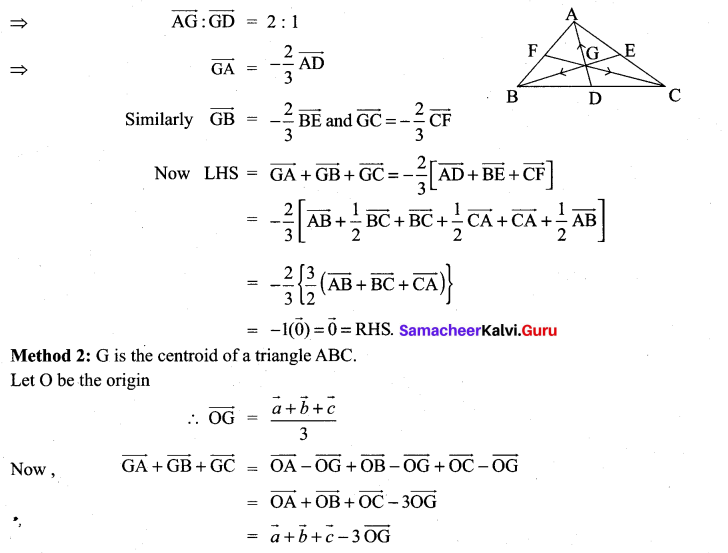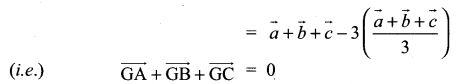Question 11.
Let A, B and C be the vertices of a triangle. Let D, E and F be the midpoints of the sides BC, CA, and AB respectively. Show that $$\overrightarrow{\mathrm{AD}}+\overrightarrow{\mathrm{BE}}+\overrightarrow{\mathrm{CF}}=\overrightarrow{0}$$
Solution:
In ∆ABC, D, E, F are the midpoints of BC, CA and AB respectively.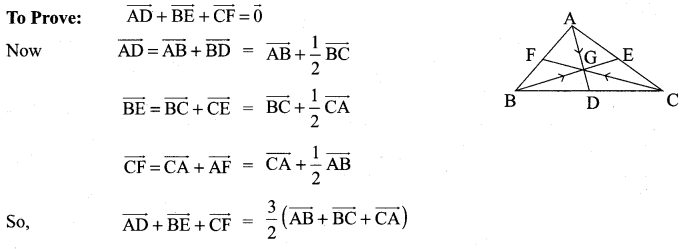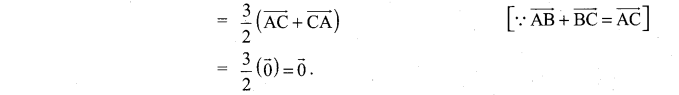Question 12.
If ABCD is a quadrilateral and E and F are the midpoints of AC and BD respectively, then prove that $$\overrightarrow{\mathbf{A B}}+\overrightarrow{\mathbf{A D}}+\overrightarrow{\mathbf{C B}}+\overrightarrow{\mathbf{C D}}=4 \overrightarrow{\mathrm{EF}}$$
Solution:
ABCD is a quadrilateral in which E and F are the midpoints of AC and BD respectively.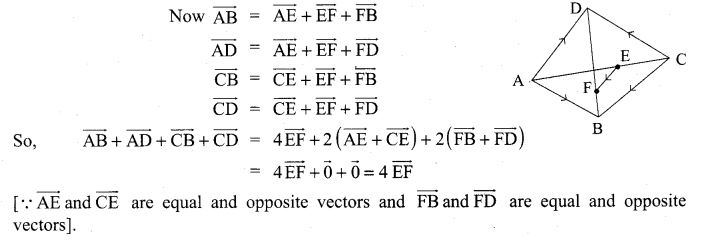### Samacheer Kalvi 11th Maths Solutions Chapter 8 Vector Algebra – I Ex 8.1 Additional Problems

Question 1.
Shown that the points with position vectors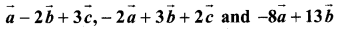are collinear.
Solution:
To prove the points P, Q, R are collinear we have to prove that
$$\overrightarrow{\mathrm{PQ}}$$ = t $$\overrightarrow{\mathrm{PR}}$$ where t is a scalar.
Let the given points be P, Q, R.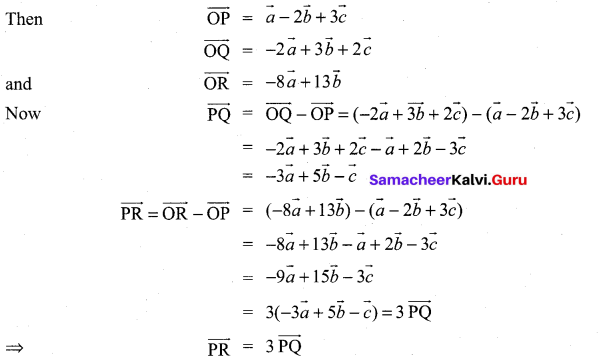So, the points P, Q, R are collinear (i.e,) the given points are collinear.

Question 2.
If ABC and A’B’C’ are two triangles and G, G’ be their corresponding centroids, prove that $$\overrightarrow{\mathrm{AA}^{\prime}}+\overrightarrow{\mathrm{BB}^{\prime}}+\overrightarrow{\mathrm{CC}^{\prime}}=3 \overrightarrow{\mathrm{GG}}$$
Solution:
Let O be the origin.
We know when G is the centroid of ∆ ABC,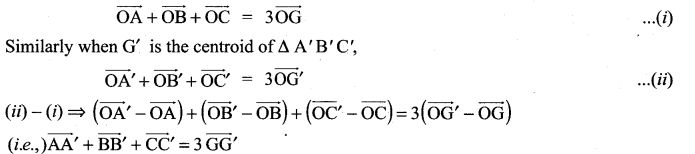Question 3.
Prove using vectors the mid-points of two opposite sides of a quadrilateral and the mid-points of the diagonals are the vertices of a parallelogram.
Solution:
ABCD is a quadrilateral with position vectors
OA = $$\vec{a}$$, OB = $$\vec{b}$$, OC = $$\vec{c}$$ and OD = $$\vec{d}$$
P is the midpoint of BC and R is the midpoint of AD.
Q is the midpoint of AC and S is the midpoint of BD.
To prove PQRS is a parallelogram. We have to prove that $$\overrightarrow{\mathrm{PQ}}=\overrightarrow{\mathrm{SR}}$$